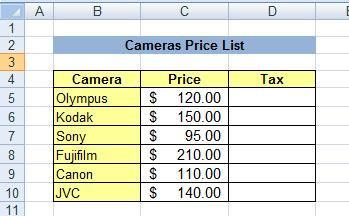Hide

# Examples of Using Absolute Reference

You can download here the worksheet from this video to practice it by yourself!

## Using absolute reference with percentages

Among the most useful usages of absolute referencing is dealing with percentages.
The percentage can relate to tax rate, interest rate, discount, bonus etc.

The percentage should be written in a cell outside the table of data. When later relating to that cell in a formula, use absolute referencing.

Let's use the example from the video above, and calculate the tax of the cameras in the following table:If we assume a tax rate of 8%, the steps that should be taken are as follows:

1. Write the tax in a cell outside the table. Let’s assume you wrote it in cell H5.
2. Inside cell D5 write the following formula:
=C5*H5 (multiplying the camera’s price by the tax rate)
3. Before hitting the Enter key, press the F4 key on the keyboard. The formula will now look like this:
=C5*\$H\$5
The meaning of this is that when the formula will be copied to all the other cells, it will multiply all the different values (the cameras prices) with cell H5 only.
4. After you have pressed the F4 key, you can now press Enter. The calculated tax will now appear in that cell.
5. Click cell D5 again, and drag it downwards with the fill handle for all other cameras.

A formula that will give you immediately the cameras final price (including tax) will be:
=C5*(1+\$H\$5)